# The plane truss is subjected to a load as shown in Figure 4. Take E = 200 GPa and cross sectional areas of members 1, 2...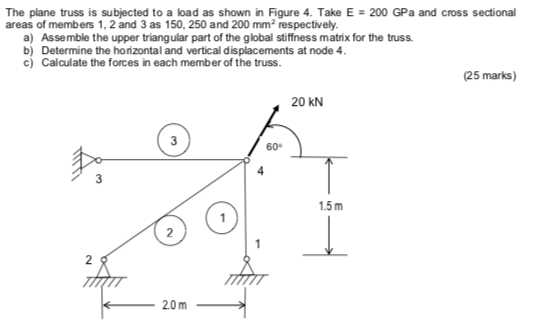The plane truss is subjected to a load as shown in Figure 4. Take E = 200 GPa and cross sectional areas of members 1, 2 and 3 as 150, 250 and 200 mm2 respectively a) Assemble the upper triangular part of the global stiffness matrix for the truss b) Determine the horizontal and vertical displacements at node 4 c) Calculate the forces in each member of the truss. (25 marks) 20 kN 3 60° 4 1.5m 2 2 20m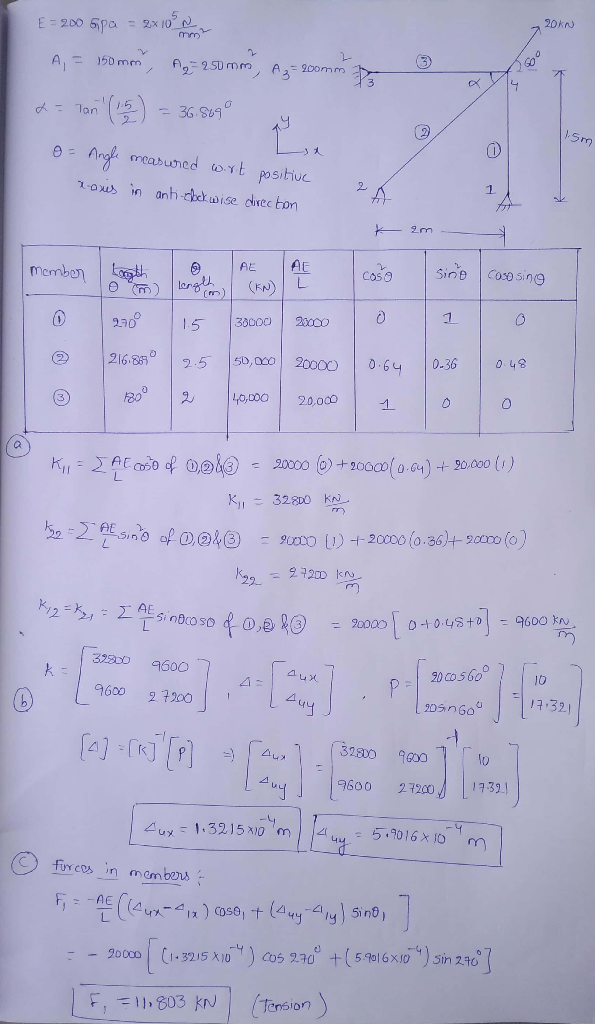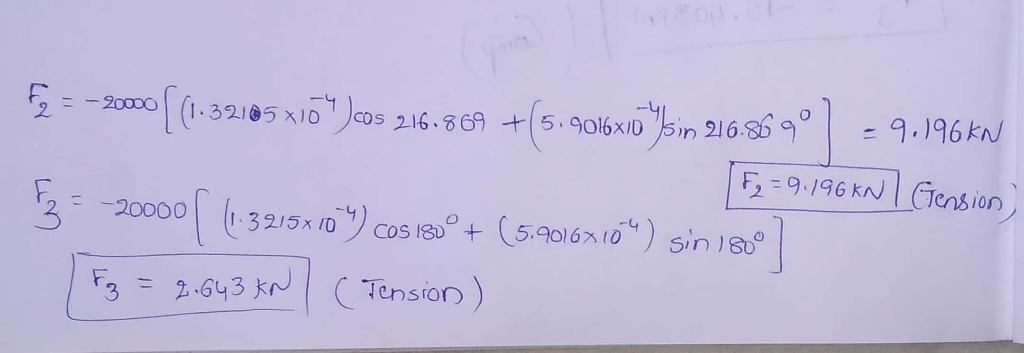#### Earn Coin

Coins can be redeemed for fabulous gifts.

Similar Homework Help Questions
• ### Question 4 The plane truss is subjected to a load as shown in Figure 4. Take E = 200 GPa and cross sectional areas of m...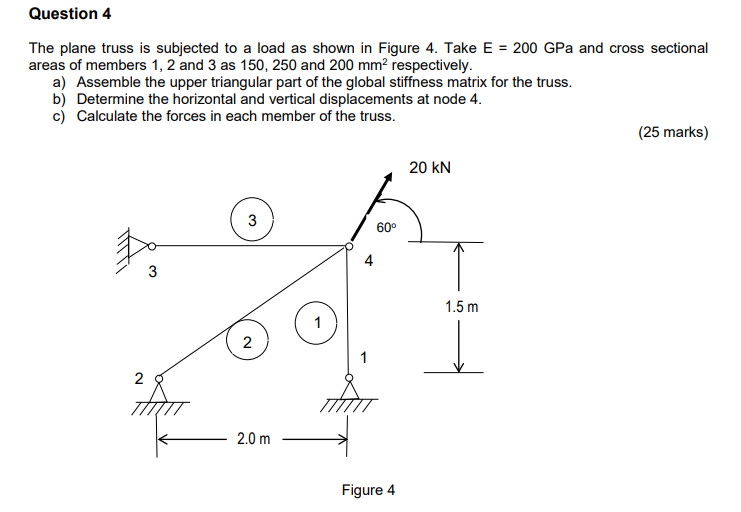Question 4 The plane truss is subjected to a load as shown in Figure 4. Take E = 200 GPa and cross sectional areas of members 1, 2 and 3 as 150, 250 and 200 mm2 respectively a) Assemble the upper triangular part of the global stiffness matrix for the truss. b) Determine the horizontal and vertical displacements at node 4. c) Calculate the forces in each member of the truss. (25 marks) 20 kN 3 600 4 3 1.5m...

• ### 2. For the pin-jointed truss shown in Figure Q2.1 applied at node 4. The Young's modulus E(GPa) is the same for...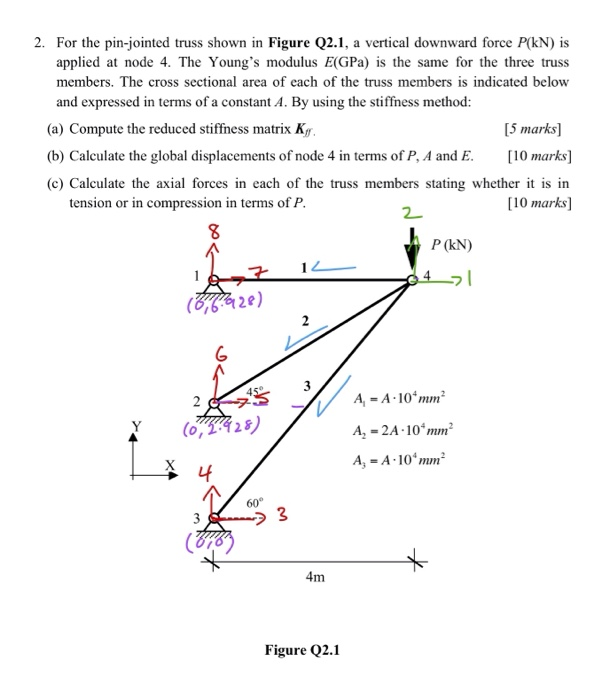2. For the pin-jointed truss shown in Figure Q2.1 applied at node 4. The Young's modulus E(GPa) is the same for the three truss vertical downward force P(kN) is a members. The cross sectional area of each of the truss members is indicated below and expressed in terms of a constant A. By using the stiffness method: (a) Compute the reduced stiffness matrix Kg [5 marks [10 marks (b) Calculate the global displacements of node 4 in terms of P,...

• ### SAN4701 JAN/FE8 2017 QUESTION 1 1 is having a hinge support at 1 and a roller support at 2. (E - 200 GPa) for all the m...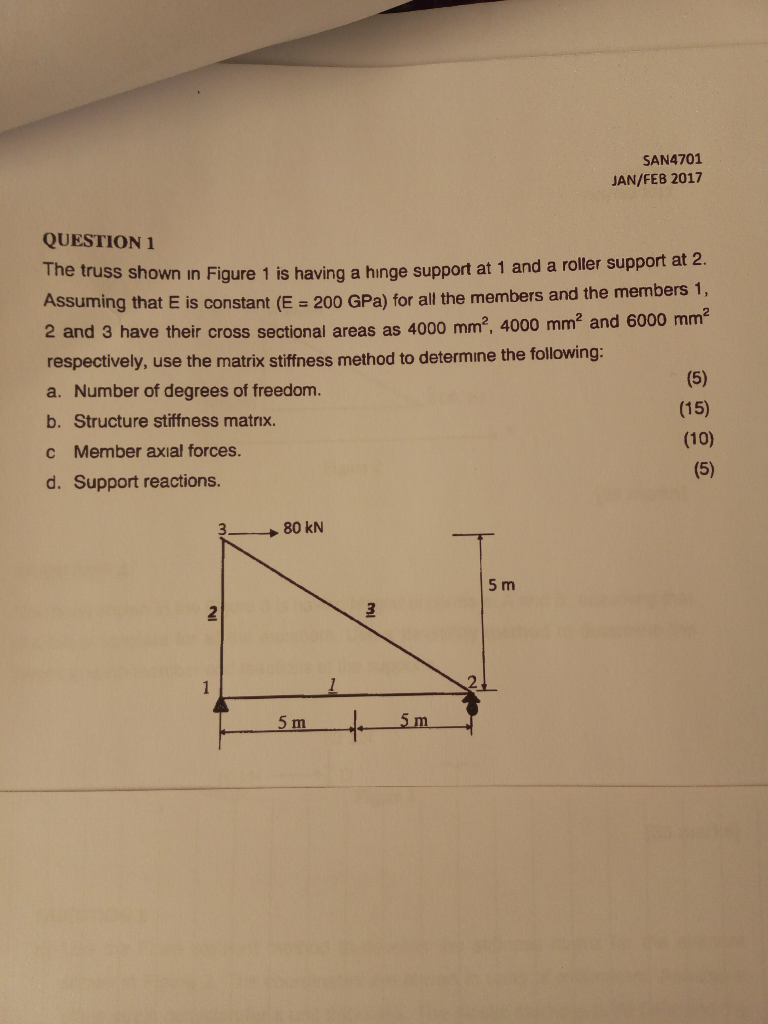SAN4701 JAN/FE8 2017 QUESTION 1 1 is having a hinge support at 1 and a roller support at 2. (E - 200 GPa) for all the members and the members 1, d 3 have their cross sectional areas as 4000 mm2, 4000 mm2 and 6000 mm2 The truss shown in Figure Assuming that E is constant respectively, use the matrix stiffness method to determine the following Number of degrees of freedom. a. (15) b. Structure stiffness matrıx. (10) c Member...

• ### Question 5 The members of the truss shown are made of steel and have the cross-sectional areas shown. Use the work e...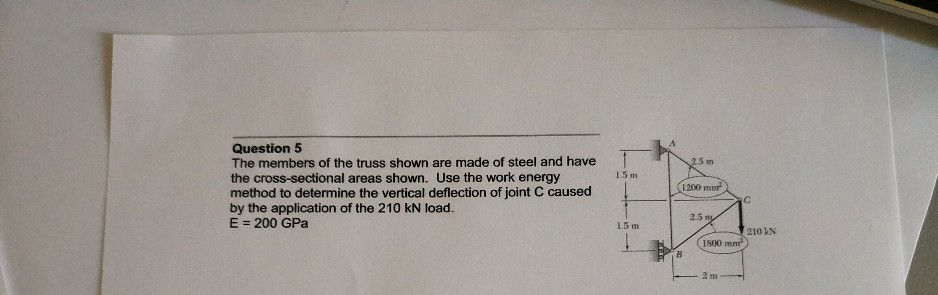Question 5 The members of the truss shown are made of steel and have the cross-sectional areas shown. Use the work energy method to determine the vertical deflection of joint C caused by the application of the 210 kN load. E = 200 GPa 15 m 1200 L5 m 210 kN 1800 Question 5 The members of the truss shown are made of steel and have the cross-sectional areas shown. Use the work energy method to determine the vertical deflection...

• ### The plane truss shown in Figure is composed of members having a square 15 mm ×...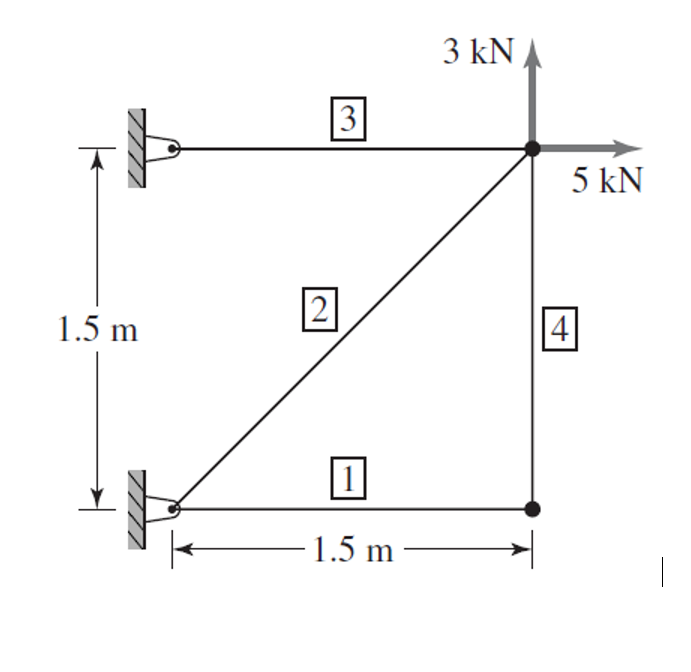The plane truss shown in Figure is composed of members having a square 15 mm × 15 mm cross section and modulus of elasticity E = 69 GPa. a. Assemble the global stiffness matrix. b. Compute the nodal displacements in the global coordinate system for theloads shown. c. Compute the axial stress in each element 3 kN 3 5 kN 2 1.5 m 4. 1.5 m

• ### For truss shown below a vertical load of 25 KN and Horizontal Load of 30 KN...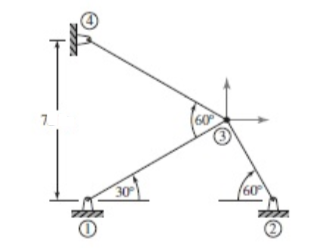For truss shown below a vertical load of 25 KN and Horizontal Load of 30 KN applied at Node 3 ( Use FEM Nodal displacement, Direct stiffness method) 1). Calculate clearly the member length and distance between members A = 5 x 10^-4 m^2 and E = 200 GPa 2). Determine the member and global stiffness matrix and show the calculation fot Sinθ and Cosθ clearly 3). determine the displacement and member forces All Load and dimensions are in meter...

• ### Figure Q5(a) shows a plane truss supported by a horizontal spring at the top node. The...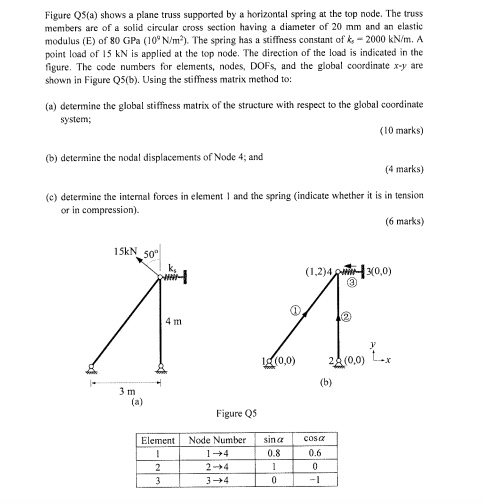Figure Q5(a) shows a plane truss supported by a horizontal spring at the top node. The truss members are of a solid circular cross section having a diameter of 20 mm and an elastic modulus (E) of 80 GPa (10° N/m2). The spring has a stiffness constant of k-2000 kN/m. A point load of 15 kN is applied at the top node. The direction of the load is indicated in the figure. The code numbers for elements, nodes, DOFS, and...

• ### Problem 2: The figure below shows a two-member plane truss supported by a linearly elastic spring....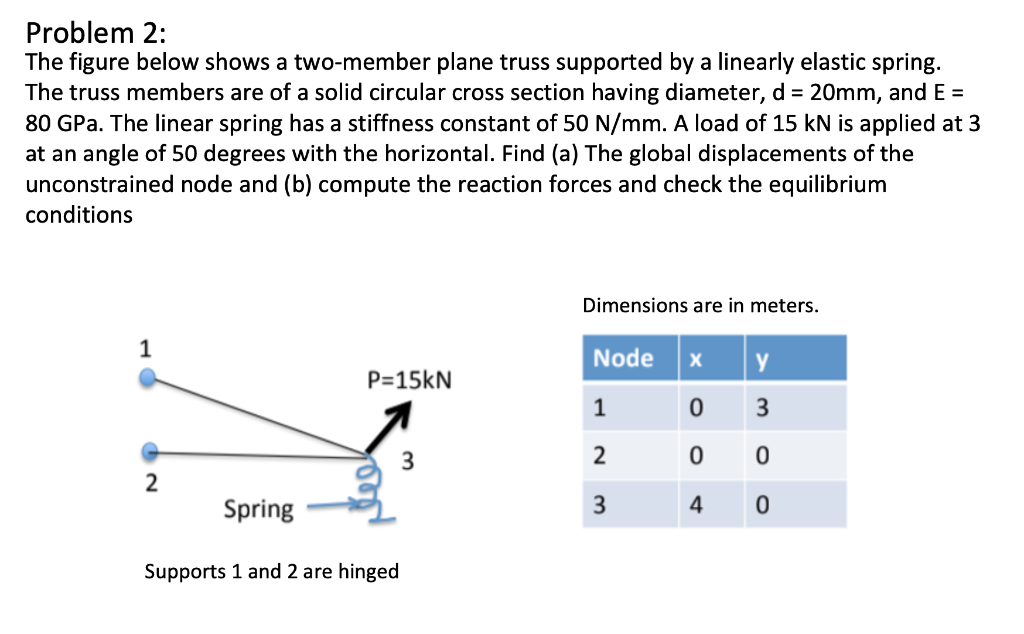Problem 2: The figure below shows a two-member plane truss supported by a linearly elastic spring. The truss members are of a solid circular cross section having diameter, d = 20mm, and E = 80 GPa. The linear spring has a stiffness constant of 50 N/mm. A load of 15 kN is applied at 3 at an angle of 50 degrees with the horizontal. Find (a) The global displacements of the unconstrained node and (b) compute the reaction forces and...

• ### two-member truss is subjected to a load P 8000 N. Me mber 1-2 is 400 mm...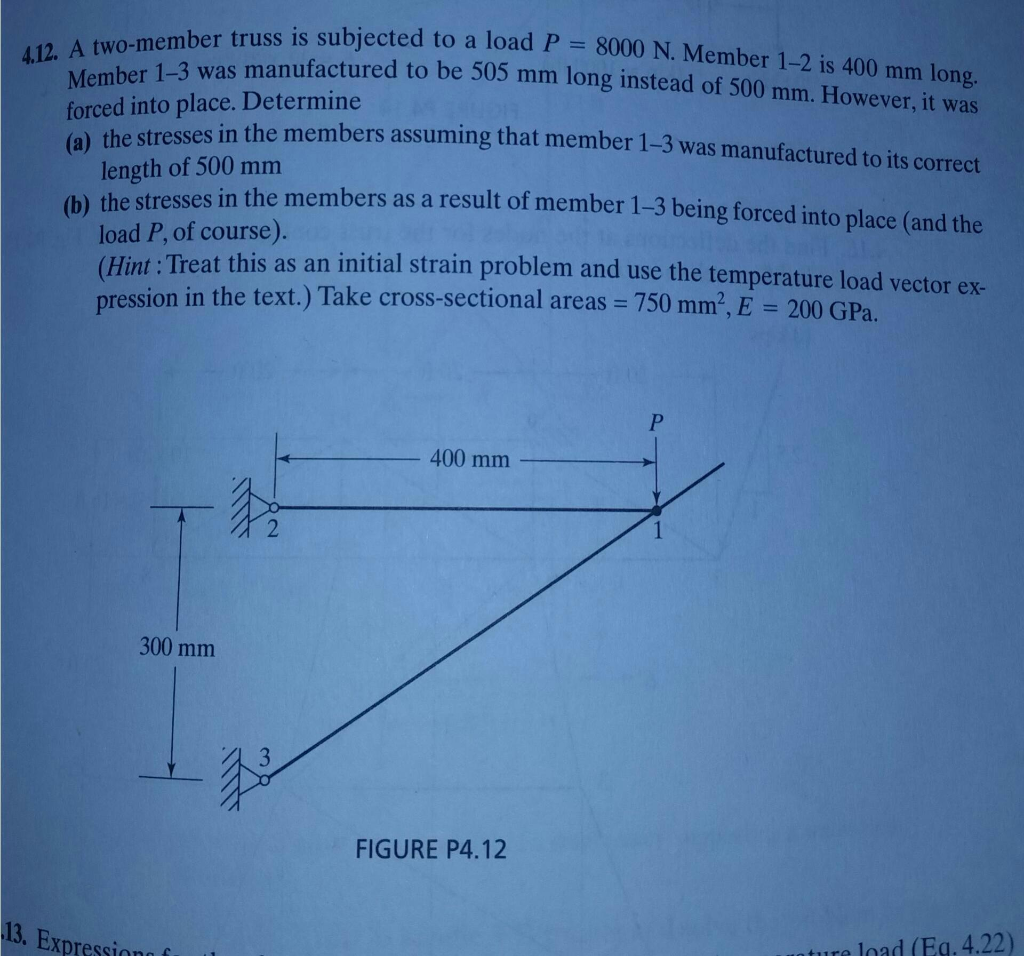two-member truss is subjected to a load P 8000 N. Me mber 1-2 is 400 mm long. 4.12. A ember 1-3 was manufactured to be 505 mm long instead of 500 mm. However, it was forced into place. Determine stresses in the members assuming that member 1-3 was manufactured to its correct (a) the length of 500 mm load P, of course). pression in the text.) Take cross-sectional areas 750 mm2, E 200 GPa (b) thestresses in the members as...

• ### 15 m B- Question 3: Each member of the truss shown is made of steel (E- 2 1 0 GPa ) and has a cro...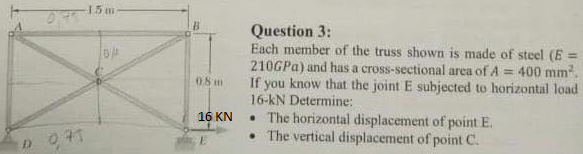15 m B- Question 3: Each member of the truss shown is made of steel (E- 2 1 0 GPa ) and has a cross-sectional area of A If you know that the joint E subjected to horizontal load 16-kN Determine: .The horizontal displacement of point E . The vertical displacement of point C. 400 mm2 0.8m 16 KN 15 m B- Question 3: Each member of the truss shown is made of steel (E- 2 1 0 GPa )...